# Problems of the surface area

#### Number of problems found: 479

• PipeHow long is the pipe with an outside diameter of 0.87 m if his coloring consumed 10 kg of color. 1 kg of color coverage is 6 m2.
• Cube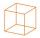Calculate the surface cube with edge 11 dm.
• SphereThe surface of the sphere is 12100 cm2, and the weight is 136 kg. What is its density?
• Rectangular fieldOne dimension of the rectangular field is 56 m greater than second dimension. If each side of the rectangle increases by 10 m, increases the surface field is 1480 m2 . Determine dimencions of the field.
• Special cube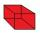Calculate the edge of cube, if its surface and its volume is numerically equal number.
• CubeThe sum of lengths of cube edges is 57 cm. What is its surface and volume?
• Observatory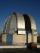Observatory dome has the shape of a hemisphere with a diameter d = 20 m. Calculate the surface.
• Rotating cone IICalculate area of surface of rotating cone with base radius r=19 cm and height h=9 cm.
• KitchenKitchen roller has a diameter 70 mm and width of 359 mm. How many square millimeters roll on one turn?
• Sphere slicesCalculate the volume and surface of a sphere if the radii of parallel cut r1=31 cm, r2=92 cm, and its distance v=25 cm.
• CapJesters hat is shaped by a rotating cone. Calculate how much paper is needed to the cap 54 cm high when the head circumference is 47 cm.
• Aquarium II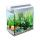Calculate how much glass we need to build an aquarium with a rectangular shape with base 70 cm × 70 cm and a height of 70 cm, if the waste is 2%. Aquarium haven't top glass.
• Concrete pipe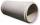Concrete pipe is cylindrical with an inner diameter 110 cm and outer 120 cm. Calculate the surface of the concrete pipe, if it is 9 m long.
• RollerCylinder shell has the same content as one of its bases. Cylinder height is 15 dm. What is the radius of the base of the cylinder?
• Cylinder - A&VThe cylinder has a volume 1287. The base has a radius 10. What is the area of the surface of the cylinder?
• Cylinder - basicsCylinder with base radius r = 54 cm and height h=35 cm. Calculate: a) Area of the base
• Sphere A2VThe surface of the sphere is 241 mm2. What is its volume?
• PresentGift box has a rectangular shape with dimensions of 8×8×3 cm. Miloslav wants to cover with square paper with sides of 18 cm. How much paper left him?
• Cube wallThe surface of the first cube wall is 64 m2. The second cube area is 40% of the surface of the first cube. Determine the length of the edge of the second cube (x).

Do you have an exciting math question or word problem that you can't solve? Ask a question or post a math problem, and we can try to solve it.

We will send a solution to your e-mail address. Solved examples are also published here. Please enter the e-mail correctly and check whether you don't have a full mailbox.